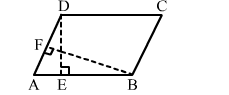# Look at the statements given below:

Question:

Look at the statements given below:
I. A parallelogram and a rectangle on the same base and between the same parallels are equal in area.
II. In a || gm ABCD, it is given that AB = 10 cm. The altitudes DE on AB and BF on AD being 6 cm and 8 cm respectively, then AD = 7.5 cm.

III. Area of a $\| \mathrm{gm}=\frac{1}{2} \times$ base $\times$ altitude.

Which is true?
(a) I only
(b) II only
(c) I and II
(d) II and IIISolution:

(c) I and II

Statement I is true, because if a parallelogram and a rectangle lie on the same base and between the same parallel lines, then they have the same altitude and therefore equal areas.
Statement II is also true as area of a parallelogram = base × height

$\Rightarrow A B \times D E=A D \times B F$

$\Rightarrow 10 \times 6=8 \times \mathrm{AD}$

$\Rightarrow A D=60 \div 8=7.5 \mathrm{~cm}$

Hence, statements I and II are true.